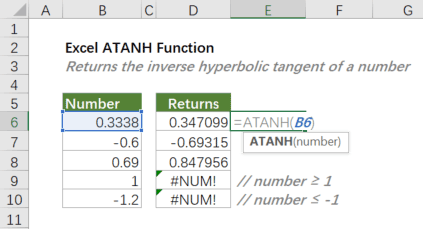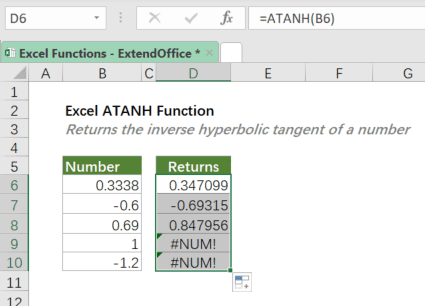## Excel ATANH Function

The ATANH function calculates the inverse hyperbolic tangent of a number.=ATANH(number)

#### Arguments

• number (required): The value for which you want to calculate the inverse hyperbolic tangent. Note: The number must be between -1 and 1.

#### Return Value

The ATANH function returns a numeric value.

#### Function Notes

• The inverse hyperbolic tangent is the value whose hyperbolic tangent is number, so the result of =ATANH(TANH(number)) is number itself.
• ATANH returns the #NUM! error if number ≤ -1 or number ≥ 1.
• ATANH returns the #VALUE! error if number is not numeric.

#### Example

To calculate the inverse hyperbolic tangent of the numbers listed in the table as shown below, please copy or enter the below formula in the top cell (D6) of the result list, and press Enter to get the result. Then select the result cell, and drag the fill handle (the small square in the lower-right corner of the selected cell) down to apply the formula to the below cells.

=ATANH(B6)Instead of a cell reference, you can type the actual number value in the formula as shown below.

=ATANH(0.3338)

#### Related functions

Excel ATAN Function

The ATAN function calculates the arctangent (inverse tangent) of a number, and returns an angle, in radians, in the range from -π/2 to π/2.

Excel ATAN2 Function

The ATAN2 function calculates the arctangent (inverse tangent) of the specified x- and y-coordinates, and returns an angle, in radians, in the range from -π/2 to π/2.

Excel ACOSH Function

The ACOSH function returns inverse hyperbolic cosine of a number.

Excel ACOTH Function

The ACOTH function returns the inverse hyperbolic cotangent of a number.

Excel ASINH Function

The ASINH function returns the inverse hyperbolic sine of a number.

Excel COSH Function

The COSH function returns the hyperbolic cosine of a number.

Excel COTH Function

The COTH function returns the hyperbolic cotangent of a hyperbolic angle.

Excel CSCH Function

The CSCH function returns the hyperbolic cosecant of an angle specified in radians.

Excel SECH Function

The SECH function calculates the hyperbolic secant of an angle in radians.

Excel SINH Function

The SINH function calculates the hyperbolic sine of a number.

Excel TANH Function

The TANH function calculates the hyperbolic tangent of a number.

### The Best Office Productivity Tools

#### Kutools for Excel - Helps You To Stand Out From Crowd

 Popular Features: Find, Highlight or Identify Duplicates  |  Delete Blank Rows  |  Combine Columns or Cells without Losing Data  |  Round without Formula ... Super VLookup: Multiple Criteria  |  Multiple Value  |  Across Multi-Sheets  |  Fuzzy Lookup... Adv. Drop-down List: Easy Drop Down List  |  Dependent Drop Down List  |  Multi-select Drop Down List... Column Manager: Add a Specific Number of Columns  |  Move Columns  |  Toggle Visibility Status of Hidden Columns  |  Compare Columns to Select Same & Different Cells ... Featured Features: Grid Focus  |  Design View  |  Big Formula Bar  |  Workbook & Sheet Manager | Resource Library (Auto Text)  |  Date Picker  |  Combine Worksheets  |  Encrypt/Decrypt Cells  |  Send Emails by List  |  Super Filter  |  Special Filter (filter bold/italic/strikethrough...) ... Top 15 Toolsets:  12 Text Tools (Add Text, Remove Characters ...)  |  50+ Chart Types (Gantt Chart ...)  |  40+ Practical Formulas (Calculate age based on birthday ...)  |  19 Insertion Tools (Insert QR Code, Insert Picture from Path ...)  |  12 Conversion Tools (Numbers to Words, Currency Conversion ...)  |  7 Merge & Split Tools (Advanced Combine Rows, Split Excel Cells ...)  |  ... and more

Kutools for Excel Boasts Over 300 Features, Ensuring That What You Need is Just A Click Away...#### Office Tab - Enable Tabbed Reading and Editing in Microsoft Office (include Excel)

• One second to switch between dozens of open documents!
• Reduce hundreds of mouse clicks for you every day, say goodbye to mouse hand.
• Increases your productivity by 50% when viewing and editing multiple documents.
• Brings Efficient Tabs to Office (include Excel), Just Like Chrome, Edge and Firefox.